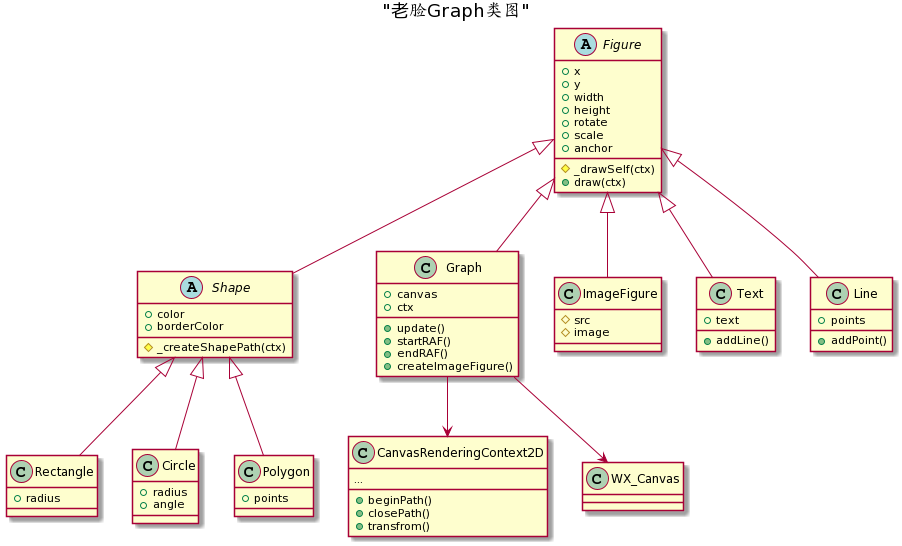Graph的工具包的介绍（1）

# 开场白

music 菊次郎的夏天

Graph工具包是什么？Js Canvas绘制究竟是什么梗？相信大家对Js Canvas绘制都很熟悉，Js Canvas绘制我们每天都会经常遇到的，但是你知道Graph工具包到底是什么吗？来随我一起看看吧。

# 简介

Graph工具包(或者叫lib)将常用的CanvasRenderingContext2D绘制方法封装成类，便于使用。

``````    ....
// 矩形大小和位置以及圆角半径：
let x = 100,y = 100,w = 100,h = 50, r = 10;
let selector = wx.createSelectorQuery();
selector.select('#canvas-id').fields({node:true,size:true})
.exec(function(result){
if(result){
let canvas = result.node;
let ctx = canvas.getContext('2d');

// 根据像素比初始化画布内存以及Context缩放：
let dpr = wx.getSystemInfoSync().pixelRatio;
canvas.width = result.width*dpr;
canvas.height = result.height*dpr;
ctx.scale(dpr,dpr);

// 基于矩形中心旋转45度
ctx.translate(50,50);
ctx.rotate(45*Math.PI/180);
ctx.translate(-50,-50);

// 绘制圆角矩形
ctx.beginPath();
ctx.moveTo(x + r, y);
ctx.lineTo(x + w - r, y);
ctx.arc(x + w - r, y + r, r, -Math.PI/2, 0);
ctx.lineTo(x + w, y + h - r);
ctx.arc(x + w - r, y + h - r, r, 0, Math.PI/2);
ctx.lineTo(x+r, y+h);
ctx.arc(x + r, y + h - r, r, Math.PI/2, Math.PI);
ctx.lineTo(x, y + r);
ctx.arc(x + r, y + r, r, Math.PI, Math.PI *1.5);
ctx.closePath();

ctx.fillStyle= "some color";
ctx.fill();
}
});
....
``````

Graph的目的在于，将图形、文字、图片等封装成一个一个的类，通过设置类的属性即可得到对应的图形以及图形变换，减少了代码编写，也不必知道过多的计算细节。

``````    const graphLib = require('../some path/graph.min.js');
....
// 矩形大小和位置以及圆角半径：
let x = 100,y = 100,w = 100,h = 50, r = 10;
let selector = wx.createSelectorQuery();
selector.select('#canvas-id').fields({node:true,size:true})
.exec(function(result){
if(result){
let canvas = result.node;
// 新建一个Graph类：
let graph = new graphLib.Graph(canvas,{
canvasWidth:result.width,
canvasHeight:result.height
});
// 新建一个Rectangle
let rrect = new graphLib.shape.Rectangle({
x : 100, y : 100 , width:100 , height:100,
color:'some color'
});

// 将图形添加到Graph中：

// 刷新Graph得到绘制结果：
graph.update();
}
});
....
``````

# Graph基本概念

## 概念

• X ：左上角x坐标
• Y ：左上角y坐标
• Width ：图形绘制区域的宽度
• Height ：图形绘制区域的高度

• Draw() ： 调用后即可绘制出这个类定义的图形。

``````    var figure = new Figure(); // 创建一个图形对象
// 这个图形所在位置的左上角坐标为 (50,50)
figure.x = 50, figure.y = 50;
// 这个图形的大小为100x100
figure.width = 100,figure.height = 100;
// 调用方法绘制它：
figure.draw();
``````

``````
// 让这个图形旋转45度，并且x轴上拉伸为原来的两倍
figure.rotate = 45;
figure.scaleX = 2;
// 调用方法绘制它：
figure.draw();
``````

``````    var rect = new Rectangle();// Rectangle是一个绘制矩形的类
var circle = new Circle();//绘制圆形的类

// 将圆形加入到矩形中，成为矩形的子图形：
.... // 设置好各自大小和位置
// 开始绘制一个矩形，它内部会绘制一个圆形：
rect.draw();
``````

## Graph的设计

Graph工具包中最基础的类，就叫`Figure`，它定义了一个图形可能拥有的一些属性，比如坐标、大小、子Figure的List等，并且还进行一些绘制规则的设定以及基本的计算（转换矩阵的计算，顶点变换后的世界坐标计算等），但`Figure`并不具体负责绘制，可以视为一个抽象类(但JS里没有这个概念)，所有具体的绘制都需要子类继承它后复写方法规定。比如一个矩形，图片，文字等，都是Graph中的一个类：## 关于Graph类

Graph工具包中有一个类叫做`Graph`，这个类是一个最顶层的`Figure`，它并不具备自身绘制功能（如果不把清空整个画布看成绘制的话）。

# 结束语